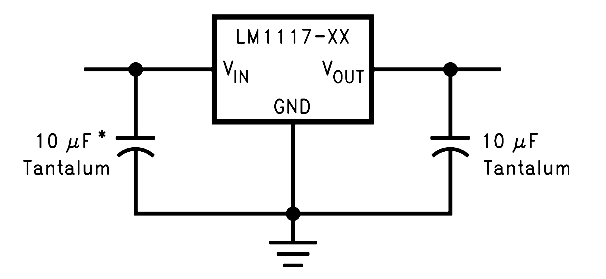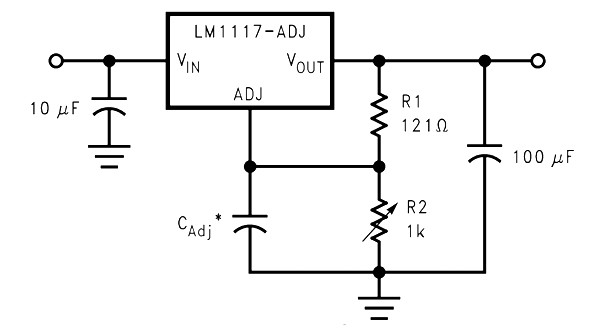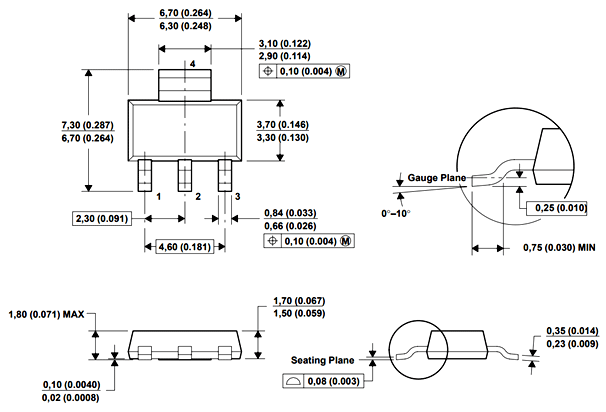# LM1117 Linear Voltage Regulator

[Click the image to enlarge it]

### Pin Configuration

 Pin Number Pin Name Description 1 Adjust/Ground This pins adjusts the output voltage, if it is a fixed voltage regulator it acts as ground 2 Output Voltage (Vout) The regulated output voltage set by the adjust pin can be obtained from this pin 3 Input Voltage (Vin) The input voltage which has to be regulated is given to this pin

### Features

• Fixed/Adjustable 3-terminal Linear voltage regulator
• Fixed Voltage type: 1.8V, 2.5V, 3.3V and 5V
• Variable Voltage range: 1.25V to 13.8V
• Output current is 800mA
• In-built Current Limiting and thermal protection.
• Operating junction temperature is 125°C
• Available in To-220, SOT223, TO263 Package

Note: Complete Technical Details can be found at the LM1117 datasheet given at the end of this page.

### Alternative Voltage Regulators

LM7805, LM7806, LM7809, LM7812, LM7905, LM7912, LM117V33, XC6206P332MR.

LM317

### Where to use LM1117 IC

Just like the famous 7805 and LM317, the LM1117 is another Linear Voltage regulator. It is known for its small form factor since it is available as a DCY Package (SMD Component). There are many types of LM1117, the fixed types provide a fixed output voltage of 1.8V, 2.5V, 3.3V or 5V and the variable voltage regulator can have a variable voltage from 1.25V to 13.8V.

So if you are looking for a SMD component voltage regulator then this IC might be the right choice for you.

### How to use LM1117 IC

Using the LM1117 is pretty much straight forward. If it a fixed voltage regulator just power the IC though the Vin pin and the regulated output can be obtained in the Vout pin. The Adj/Ground pin in this case acts only as a ground pin and is grounded. Also a capacitor can be added at the output side to filter out the noise. The circuit diagram for a fixed output regulator is show belowFor an Adjustable type voltage regulator we need two external resistors to decide the output voltage of the Regulator. A reference circuit diagram is shown below, where the resistors R1 and R2 decides the output voltage of the regulator. The capacitor CAdj is an optional component which can be added to improve ripple rejection if required. The other two capacitors are to filter the input and output noise respectively.The formulae to calculate the output voltage of the regulator is given below. Select the value of R1 and R2 based on the output voltage required for your project. Keep in mind that the value of R1 should be less than 1k. You can use a variable resistor at R2 if you want to vary the voltage in real time.

VOUT = 1.25 × (1 + (R2/R1))

With the ability to be used as a fixed voltage or a variable voltage regulator the LM1117 often finds its application in battery charging circuit and can also be designed to provide negative voltage if required. Refer the datasheet at the end of this page to find more application circuits for this IC.

### Applications

• Used for Positive voltage regulations
• Variable power supply
• Current limiting circuits
• Reverse polarity circuits
• Commonly used in Desktop PC, DVD and other consumer products
• Used in motor control circuits

### 2D – Model of LM1117 (DCY Package)